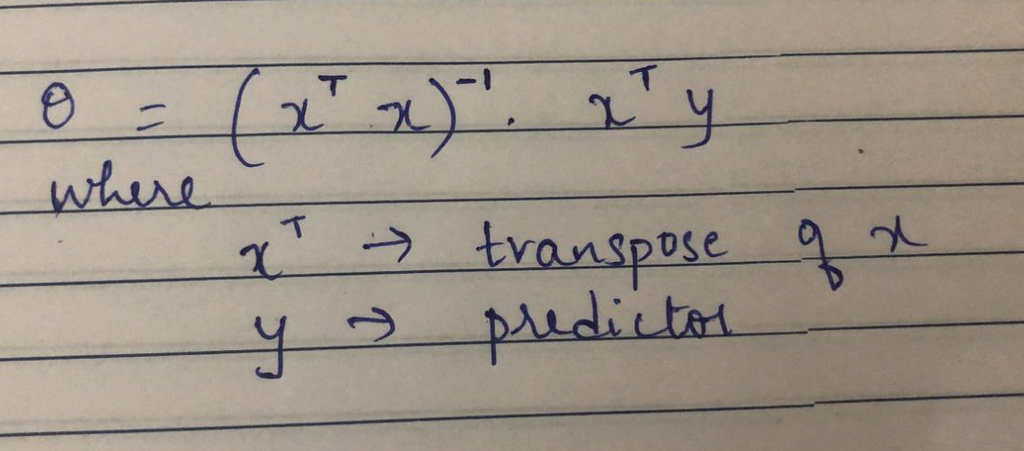## PublicationMachine Learning

# Normal Equation in Linear Regression

Last Updated on June 25, 2020 by Editorial Team

#### Machine Learning

Gradient descent is a very popular and first-order iterative optimization algorithm for finding a local minimum over a differential function. Similarly, the Normal Equation is another way of doing minimization. It does minimization without restoring to an iterative algorithm. Normal Equation method minimizes J by explicitly taking its derivatives concerning theta j and setting them to zero.

Example:

Below is a data-set to predict house price:

House Features:

Predictor:

Calculation:

`import numpy as np`
`x = np.array([[1, 2104, 5, 1, 45],        [1, 1416, 3, 2, 40],        [1, 1534, 3, 2, 30],        [1, 852, 2, 1, 36]])`
`y = [460, 232, 315, 178]`
`x_transpose = x.transpose()`
`x_transpose_x = np.dot(x_transpose, x)`
`x_transpose_y = np.dot(x_transpose, y)`
`theta = np.dot(x_transpose_x_inverse, x_transpose_y)theta`

### Gradient Descent Vs Normal Equation

• It requires to choose the value of Alpha.
• It requires many iterations.
• It works well when n (no. of data-set) is large.

#### Normal Equation

• It does not need to choose the value of Alpha.
• It doesn’t require iteration.
• It requires to calculate the inverse of transpose of x.
• It is slow if n (data-set) if very large.

### Linear Regression with Normal Equation

Import libraries:

`from numpy import genfromtxtimport numpy as npfrom matplotlib import pyplot as pltfrom sklearn.model_selection import train_test_splitfrom sklearn import linear_modl`

`data = genfromtxt('portland.csv', delimiter=',')`
`area = data[:, 0]`
`rooms =data[:, 1]`
`price = data[:, 2]`

Visualize The Area against the Price:

`def fitTheta(feature, theta):`
`return np.dot(theta, feature)`
`def visualiseFeature(feature, featureLabel, thetaVal=None):`
`fig = plt.figure(figsize=(20, 10))        plt.rcParams.update({'font.size': 10})        plt.xlabel(featureLabel, fontsize=15)        plt.ylabel("Price", fontsize=15)        plt.scatter(feature, price, color="red", s=75)        if(thetaVal):            thetaFit = fitTheta(feature, thetaVal)                plt.plot(feature, thetaFit)`

Visualize the Number of Rooms against the Price of the House:

`visualiseFeature(rooms, "Number of Rooms")`

Here, the relationship between the Number of Rooms, and the Price of the House, appears to be Linear.

Define Feature Matrix, and Outcome/Target Vector:

`X_data = data[:, 0:2] #Feature Matrix`
`y = data[:, 2] #Outcome Vector`

Calculate Cost Function:

`def getMSE(feature, thetaRange):    costMatrix = np.repeat(price, thetaRange.shape).reshape(price.shape, thetaRange.shape)        costs = np.dot(np.asmatrix(feature).T, np.asmatrix(thetaRange)) - costMatrix        MSE = (np.array((np.sum(costs, 0)))**2)/(2*price.shape)        return np.array(MSE)`

Visualize Cost Function:

`def visualiseLoss(feature, featureName, startInterval, endInterval, stepSize=0.5, thetaVal=None):    thetaRange = np.arange(startInterval, endInterval, stepSize)        Loss = getMSE(feature, thetaRange)        fig = plt.figure(figsize=(20, 10))        plt.title("Loss Function for the Feature: {}".format(featureName), fontsize=25)        plt.ylabel("Cost Function, J(Θ)", fontsize=15)        plt.xlabel("Weight(s), Θ", fontsize=15)        plt.plot(thetaRange, Loss, zorder=1)        if(thetaVal):            thetaLoss = getMSE(feature, np.array(thetaVal).reshape(1, 1))                plt.scatter(thetaVal, thetaLoss, marker="x", linewidth=5, color="red", s=200, zorder=2)                plt.annotate("Theta = {}".format(thetaVal), (thetaVal, thetaLoss), fontsize=25)`
`visualiseLoss(area, "Area", -500, 800)`

`visualiseLoss(rooms, "Rooms", -40000, 250000)`

### Split Data

`X_data = data[:, 0:2] #Feature Matrix`
`y = data[:, 2] #Outcome Vector`
`X = np.c_[np.ones(X_data.shape), X_data]`
`X_train, X_test, y_train, y_test = train_test_split(X, y, test_size=0.2)`

### Normal Equation

`firstTerm = np.linalg.inv(np.dot(X_train.T, X_train))`
`secondTerm = np.dot(X_train.T, y_train)`
`Theta = np.dot(firstTerm, secondTerm)`

Visualize:

`visualiseLoss(area, "Area", -500, 800, thetaVal=Theta)`
`visualiseFeature(area, "Area (Square Feet)", thetaVal=Theta)`

### Prediction using Normal Equation theta value

`normal_predictions = np.dot(X_test, Theta)`
`normal_predictions`

### Prediction using Linear Regression

`reg = linear_model.LinearRegression()`
`reg.fit(X_train, y_train)`
`sk_predictions = reg.predict(X_test)`
`sk_predictions`

Here, the predictions from the Normal Equation and Linear Equation are the same.

### Normal Equation Non-Invertibility

A squared matrix that does not have an inverse a matrix is singular if and only if it is determined is zero.

Example:

`import numpy as np`
`A = [[2, 6],`
`[1, 3]]`

The inverse of Matrix:

`inverse_A = np.linalg.inv(A)`
`inverse_A`

Error from Numpy:

Problem due to Non-Invertibility:

• Redundant features
• Too many features

How to solve if there are too many features?

• Delete some features of use Regularization

### Conclusion

Gradient Descent gives one way to minimizing J. Normal Equation is another way of doing minimization. It does minimization without restoring to an iterative algorithm. But, Normal Equation is very slow if the data-set size is very largeNormal Equation in Linear Regression was originally published in Towards AI — Multidisciplinary Science Journal on Medium, where people are continuing the conversation by highlighting and responding to this story.

Published via Towards AI

## Comment (1)

1.May 5, 2021

Code below the dataset is incorrect and it doesnt work when we define the x_transpose_x_inverse